# Expression Engine Guide

In this tutorial you will learn how to use the expression engine built into MobiFlight. You will learn tips and tricks and you will see that you can unlock a lot of new potential to your configurations.

### Introduction

MobiFlight is programmed to be particularly user-friendly. In order to be able to adapt the values read by the simulator to instruments, steppers, servos or digital displays, the possibility was created to adapt these values using formulas.

The NCALC module is the basis for the calculations in MobiFlight. Those interested can read it here:

https://github.com/ncalc/ncalc/wiki

This guide is not about exhausting the last possibilities of the calculations, but rather showing the possibilities of converting values from the simulator into a desired form.

The following basic rules apply to all formulas that are processed in MobiFlight:

• a formula never contains spaces
• the decimal point for numbers is always a point (.)
• the separator for arguments is always the comma (,), never the semicolon (;)

## Simple Calculations

A config is required so that formulas can be entered. Formulas can only be entered for outputs (values that are output by the simulator).

To practice with formulas, we first need a config in the Outputs tab, which is to be given a name. On the right in this line is the field with the 3 dots under the heading Edit.The \$ symbol in then transform field always refers to the offset that has been selected in the basic settings. In this case it would be the offset 0x0000. This offset is always specified as the standard by MobiFlight, unless another offset is entered or selected from the preset list.

Now it can happen that I don’t need an offset at all, but simply want a value in MobiFlight with which I want to continue calculating, or, as here, by doing simple calculations that do not refer to a simulator value

If no simulator value is desired, the \$ sign can be removed from the transform line and I simply enter a 1 or any other number that I would like to refer to later.
More on this in Part 5.

So it is possible that I "create" my own values with a transform line.

The result of a transform is always an integer value, never a float or a string! Transform cannot process strings either. If a float value is required, it can be obtained by multiplication by pulling the relevant decimal places forward by multiplying by 10, 100, 1000 etc.

### The Basic Arithmetic

Assuming the value of an offset is 2020. Then I can edit or change it using the 4 basic arithmetic operations.

In order to be able to show this with an example number, the offset 0x0240 (Zulu Year in FS) was used.

#### Examples:

Transform: \$+100
Result      : 2020+100 = 2120Transform: \$-100
Result      : 2020-100=1920Transform: \$/5
Result      : 2020/5=404Transform: \$*3.5
Result      : 2020*3.5=7070Combinations of the basic arithmetic operations are also possible. The mathematical rules apply here:

• Point calculation before line calculation, i.e. multiplication / division before addition / subtraction
• What is in brackets is calculated first

#### Example:

Transform: 3+4*5
Result      : 3+4*5=23

4*5=20 + 3 = 23Transform: (3+4)*5
Result      : (3+4)*5=35Probably the most frequently used formula is the conversion of the frequency values that the simulator returns (P3D / FSX). The value read is e.g. 2280. In order to make the displayed frequency of 122.80 from this, the following is written in the transform line:

Transform: \$+10000

Result      : 1228+10000=12280The screenshot shows the conversion here. The simulator reads the value 2280 and the output value is 12280. The decimal point is then defined in the display and 122.80 appears on the display.

In the meantime there are newer versions of FSUIPC in which other offsets are used for these frequencies, where this addition is no longer necessary because the frequency is output in HzBut here, too, you can adapt the display so that it fits the corresponding 5 or 6-digit display.### Rounding Numbers

The so-called 5/4-rounding is usually used for rounding. If a decimal point (e.g. 0.5) is greater than or equal to 5, it is rounded up to the next highest number. If the decimal point is less than or equal to 4, the number is rounded down to the next lower number. So 7.6 becomes 8 and 7.3 becomes 7.

What is rounding required for? The transform line always outputs an integer as the result. Integer means something like the whole number part of a number, or also whole number. Fractions after the decimal point are not displayed, but are carried internally to the result. FSUIPC provides a number of offsets in which any amount has to be calculated. Understandable, if the numbers don't always come out in the evenings.

In order to make the values ​​more precise, it is multiplied or divided at one point or another and the result would be numbers with decimal places.

In order to have these relatively exact, they are rounded. Either up or down.

How is the rounding carried out?

The syntax is: Round(number, decimal places)

The capital "R" is important for the Round command. If the formula is written with a small "r", nothing happens or MF aborts with an error. round () also does not work. Please pay attention!

Number is any number, the content of an offset or the result of a calculation.

Decimal places indicates how many decimal places the value before the decimal point is taken into account in the calculation.

Round(7.6,0) rounds the result up to 8. This means that 0 takes into account the 1st number after the decimal point when rounding.On the other hand, a Round(7.63,1) rounds the result down to 7.6. But only 7 is displayed. How is that?The 2nd digit after the decimal place is now significant for rounding. It rounds off to 7.60.

If I write the formula like this: Round(7.63,1) * 10 I get 76 as the result.This is due to the fact that MF internally ignores 7.6 in the calculation as long as the 2nd decimal place is less than or equal to 4.

On the other hand Round(7.66,1)*10 givesThis also works with the 3rd and every subsequent decimal place.

So it is important to know what to round off as an offset (\$) or value in my calculation.

If I need the 3rd position after a comma for a value because I need this for further processing, it makes sense to multiply the value by 100 from the start and then focus on the 1st decimal place.

Round(7,643 * 100.0)has as a result 764 while

Round(7.648*100,0)

has 765 as a result.So much for the rounding of numbers and the Round command in MobiFlight.

### Formulas with Conditions – IF

If I want to influence the behavior of MobiFlight in such a way that actions are dependent on certain values, I cannot avoid setting conditions and evaluating them.

The IF command is used for this.

Syntax: if (condition, then [true], otherwise [false])

So there is a condition. If this condition applies (if it is true), what is referred to as "then" is calculated; if it does not apply (if it is false), what is called "otherwise" is calculated.

As a reminder: the \$ symbol is a placeholder for the offset that we selected above.

An example:

if(\$=1,1,0)

If the offset value (\$) is equal to 1, "then" the output value of MF should be 1, "otherwise" 0.

Granted, a simple example, but it's just meant to show the possibilities.

The (relational) operator equal (=) can also be:

=        same
>        bigger
>=      greater than or equal to
<        smaller
<=      less than or equal to
!=       not the same

In addition to the relational operators, there are also the logical operators. These are

or       ||

and     &&

An example:

if(\$>179,\$+180,\$)

translated:

if \$ is greater than 179, then \$ + 180, otherwise \$

If the offset value is smaller than 180, this value is output. If the offset value is greater than 179 (e.g. 185), 180 is added to the offset value (365). The otherwise output is not taken into account.

For example, if I want to control an LED with an offset, I also have this option if the offset value is not just 0 or 1:

Assuming the offset value changes in the range from 0 and 100 and I want to control an LED in the range 80 to 90, then this can be done as follows:

if(\$>=80&&\$<=90,1,0)

If the offset value is greater than or equal to 80 AND the offset value is less than or equal to 90, then a 1 is output, otherwise a 0.

That can now be increased.

If I want the LED to be switched on in the range 20 to 30 and in the range 80 to 90, I can enter this into a formula as follows:

if(\$>=20&&\$<=30,1,if(\$>=80&&\$<=90,1,0))

Here I have now linked two if-conditions.

The 1st condition outputs a 1 if the offset value is greater than or equal to 20 and less than or equal to 30. However, if the offset value is 85, the "then" condition is not fulfilled and MF jumps to the "otherwise" condition.

Here again there is an if that checks the range greater than or equal to 80 and less than or equal to 90. Since this condition applies, a 1 is now output here.

What happens now if the offset value is outside of these two ranges, e.g. at 55?

if 55>= 20 AND 55<= 30 (false), i.e. no 1, the "else" condition applies, which begins again with if. It is now checked whether 55> = 80 AND <= 90, which is also wrong, so no 1 is output here either.

The 2nd "else" condition takes effect, which results in a 0 as output. The LED stays off.

It is possible to combine more than two If-conditions and choose the values ​​as they are needed.

It is important to close the brackets again at the end, otherwise MF cannot perform the correct calculation.

### Formulas with Modulo

https://de.wikipedia.org/wiki/Division_mit_Rest

Modulo is basically nothing more than a division by a number that leaves a remainder as a result. What is interesting is not what comes out of the division, but the rest.

An example:

A%B = C

The modulo does not show how often B fits into A, but how big the remainder C is.

Therefore writes

20%5 = 0

and means that 20/5 = 4 with the remainder zero.

21%5 = 1

So it's nothing more than 20/5 = 4 remainder 1

If A is less than B, then C = A, because

10%16 = 10, therefore 10/16 = 0 remainder 10

The rest, i.e. C, can never be B, because then A = B and C would be 0.

10%10 = 0

So much for the theory. Why do we need something like this in MobiFlight?

The COM frequencies in the older simulators are in the 25 kHz grid.

Starting with 120,000, the next frequency would be 120.025, then 120.050, 120.075, 120.100, etc.

However, the simulator always only provides 4 digits, i.e. 120.00, 120.02, 120.050, 120.07, 120.10 etc.

If I now want to display the 3rd position in the COM devices, I take the basic frequency of the simulator, here 2000 and add the value 10000 to this and then get 12000 in the display. With the decimal definition in the display tab, this becomes 120.00.

In order to display the 6th position correctly, I use the modulo calculation.

The simulator outputs 2000, 2002, 2005, 2007, 2010. Adding 10000 each I get 12000, 12002, 12005, 12007, 12010 and with the decimal representation 120.00, 120.02, 120.05, 120.07, 120.10

For the 6th position, i.e. the 3rd position after the decimal, I use the same offset and write the following formula in the transform line:

if(\$%5=0,0,5)

If the result of the modulo calculation is 0 with 5, then 0 should be output as the last digit, otherwise 5.

If I divide the simulator value by 5, at 2000% 5 I get the result 0 and 20000 is displayed. With the value 2002 it results with modulo the value 2002% 5 = 2. So the result is not 0 and the 6th digit is displayed as 5 and I get 20025 or the correct frequency 120.025.

With this frequency grid, there is always a 5 at the end if the frequency that the simulator delivers ends on 2 or 7.

The application possibilities of the modulo calculations are very diverse and a real help.

At this point, part 1 of working with formulas ends. If you liked the guide and if you want to know more, read Part 2, which deals with the comparison of values.

Pizman82 and StephanHo for MobiFlight in October 2020

## Comparison of Values

The comparison of values always refers to the currently selected value of the offset that has been selected in the FSUIPC tab. If Transform is used, the comparison then refers to the result of Transform. In any case, the result is always an integer value.

This is based on whether the reference value (offset) is equal to (=), greater than (>), greater than or equal to (>=), less than (<), less than or equal to (<=) or not equal to (!=).

For example, if I want to set an LED when the aircraft's autopilot (AP) is below 10,000ft, I first look for the offset for that value. It is the offset 0x652E (B737 PMDG)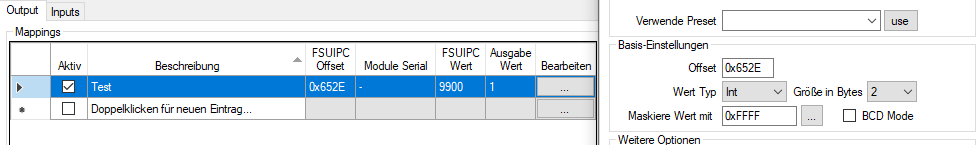Then I switch from the FSUIPC tab to the Compare tab, set the checkmark, and select smaller than (<). The value 10000 is entered in the field next to it. <=9999 also works.

Next to “set it to” a 1 is entered, and in the field next to “else set it to”  the number 0 is entered.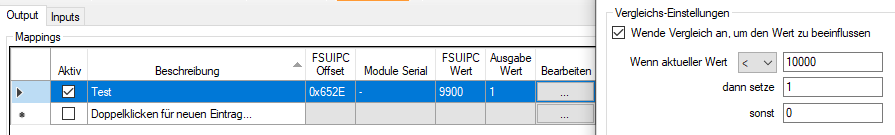As can be seen, a 1 is put out at 9900ft and this should turn on the LED, which is then selected under the Display tab.

Several formulas are possible here, which have the same result, e.g.

If >=10000 then 0 else 1 or

If <10000 then 1 else 0

If >9999 then 0 else 1

If the value in the AP is now increased to 10,000ft, the following picture appears: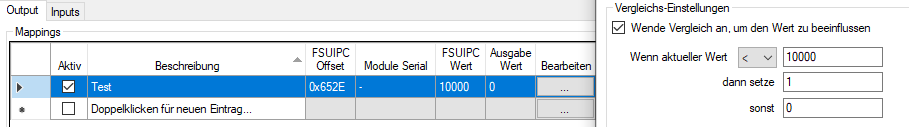The output value changes to 0, as soon as the current value reaches 10,000ft or more.

In contrast to the transform line, it is also possible to work with texts here.

Instead of 0 or 1, a text can be the output here. Of course, this depends on the settings of the Display tab. This would not be possible with a 7-segment display, but i.e. with an LCD.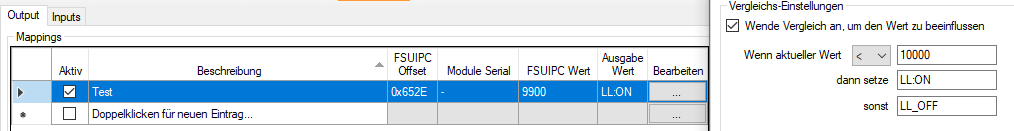For a value above 10,000ft the text changes to LL_OFF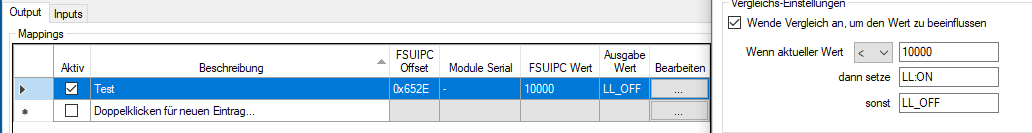With a 7-segment display, the display can virtually be deleted by entering the space bar five times for the “set it to” value,  and simply setting the “else set it to” value to the offset value (\$)

In Compare, a formula can be used instead of a number.

If a value is greater than 10000 then 1 else 0

if(\$>10000,1,0)

Strings are also possible

if(\$<=10000, ‘LL_ON’,’LL_OFF’)

This was part 2 of the guide about formulas which deals with the comparison of values. Part 3 deals with the preconditions.

## Preconditions

Preconditions are intended to ensure that configurations can only become active when the precondition selected for them is active.

In turn, this means that configurations are inactive as long as the precondition(s) are not or no longer fulfilled.

Even though the tab is called precondition, it is possible to define several preconditions.

If several preconditions are used, they are linked together, which means that they are logically put in relation to each other.

As linking possibility, the logical AND and the logical OR can be used.

For logical links, a truth table can be used for a better understanding. A truth table gives information about all the possible combinations of the input conditions and the corresponding truth value of the total statement.

truth table AND

A B C

0 0 0

1 0 0

0 1 0

1 1 1

In this truth table, the overall statement (C) is true only if the two preconditions (A) and (B) are also true.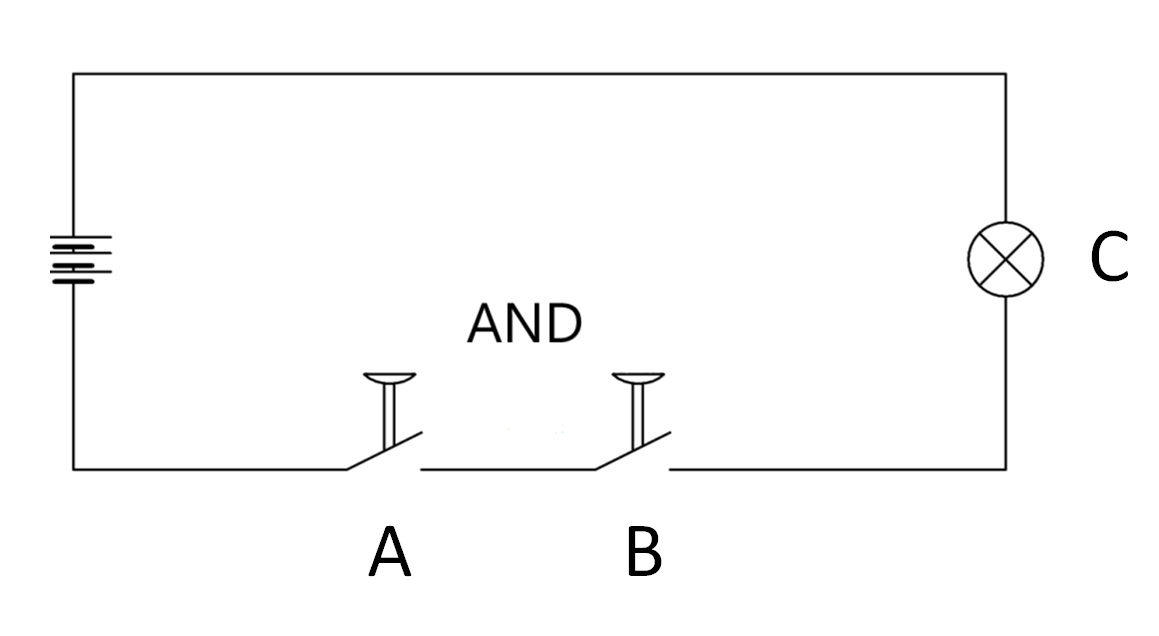truth table OR

A B C

0 0 0

1 0 1

0 1 1

1 1 1

In the truth table for the OR, the overall statement (C) is true whenever either one of the two preconditions (A) or (B) are true or both preconditions (A) and (B) are true.

If for both truth tables a switch is chosen for preconditions (A) and (B), then a downstream lamp will be lit if the overall statement (C) is true.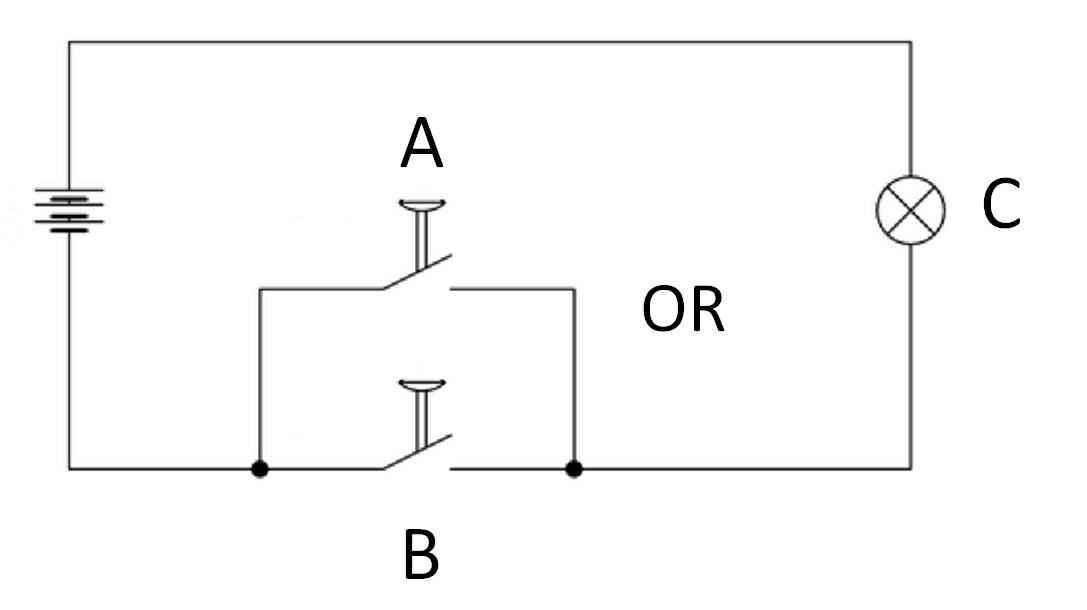In every configuration it is possible to define as many preconditions as desired or required.

It should be noted that preconditions are either AND or OR linked and are processed accordingly in sequence.

If I have precondition A AND precondition B OR precondition C,

I then check whether the condition A AND B is fulfilled or whether the condition B OR C is fulfilled.

If there is still an AND or OR after the last condition, it can be ignored, since it has no function (in a later version this will be left out, because it can lead to confusion).

Popular in this context is the representation of LEDs and displays, which should only show something if the Battery_Master_Switch is switched on.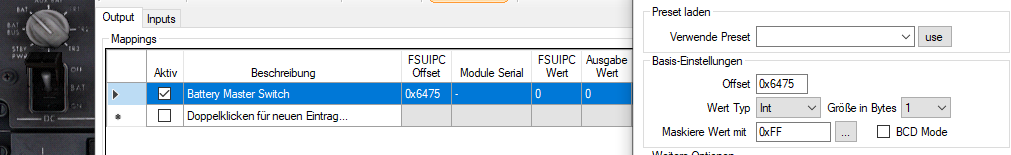In diesem Beispiel handelt es sich um den Battery_Master_Switch der PMDG B737 NGX. Bei den Standard-Flugzeugen ist dies der Offset 0x281C.

Ist der Master_Battery_Switch eingeschaltet, ändert sich der Offset: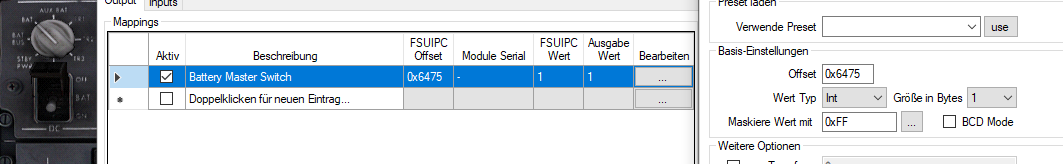Wenn eine LED leuchten soll, so wäre hier die Vorbedingung, daß der Master_Battery_Switch EINgeschaltet ist.

Für unser Beispiel nehme ich die Darstellung für die Frequenz von COM1 active. Hierzu benutze ich den Offset 0x034E, da dies der übliche Offset ist. Neuere Versionen benutzen 0x054C (siehe dort).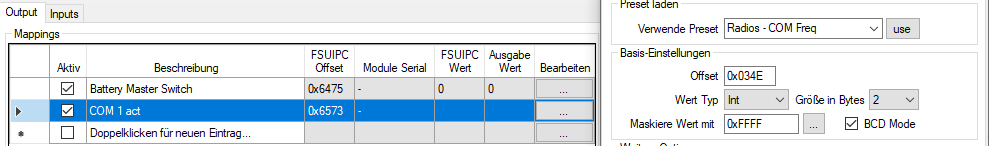Bei dieser Version ist darauf zu achten, daß die Transformzeile benutzt wird, um eine korrekte Darstellung der Frequenz zu ermöglichen.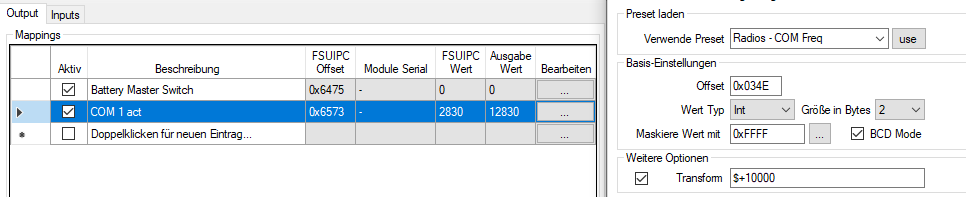Da noch keine Vorbedingung erstellt worden ist, wird diese Frequenz aber bereits auf dem Display dargestellt. Damit dies nun nicht passiert, brauche ich eine Vorbedingung, die wie folgt aussieht:

Hierzu wird der Tab VORBEDINGUNG ausgewählt. In der Liste der Vorbedingungen wird vor die erste Bedingung ein Haken gesetzt. Darunter wähle ich im Bereich „Art der Vorbedingung“ den Config Eintrag aus.

Darunter wähle ich dann aus der Liste den von mir erstellten Eintrag „Battery Master Switch“ aus und lege fest, daß der aktuelle Wert dieser Config 1 sein muß, um die Vorbedingung zu erfüllen.

Durch Anklicken von „Anwenden“ wird diese Vorbedingung übernommen. Das (AND) dahinter kann ignoriert werden, solange keine weitere Vorbedingung hinzugefügt wird. Dann kann dieser Operator auch in OR geändert werden (rechte Maustaste).

Meine Config sieht nun so aus: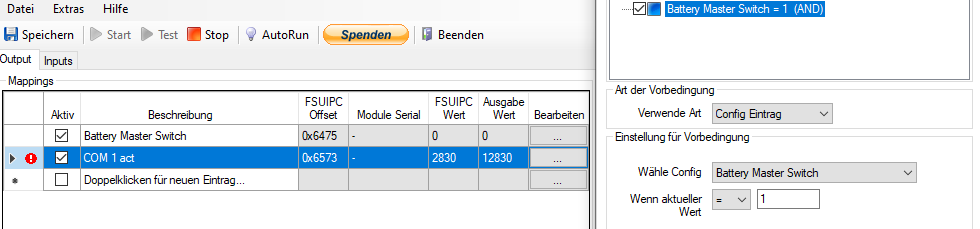Da aus dem Screenshot ersichtlich ist, daß der Battery Master Switch noch ausgeschaltet ist (FSUIPC-Wert = 0, Ausgabewert = 0) erscheint vor der Config für COM1 active eine roter Kreis mit einem Ausrufezeichen. Dies zeigt an, daß die Config derzeit nicht aktiv ist und somit keine Ausgabe auf dem Display erfolgt.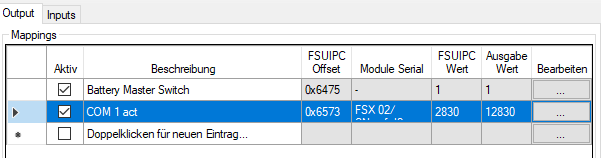Schalte ich nun den Battery Master Switch ein, wechselt der Wert seiner Config von 0 auf 1. Dies hat zur Folge, daß die Vorbedingung für Com1 act nun erfüllt ist und das Ausrufezeichen im roten Kreis vor der Config nicht mehr angezeigt wird. Somit wird die Frequenz auch auf dem Display angezeigt.

Schalte ich nun den Battery Master Switch wieder aus, muß ich feststellen, daß die Frequenz weiterhin auf meinem Display dargestellt wird.

Wie kommt das?

Wenn ich in MobiFlight etwas einschalte, dann muß ich es auch wieder ausschalten. Dies mache ich normalerweise mit einem Schalter. Da ich hier aber zum Einschalten eine Vorbedingung benutze, die NUR einschaltet, brauche ich auch eine Vorbedingung, die das Display auch wieder ausschaltet.

Hierzu nutze ich zweierlei Dinge. Einmal die Vorbedingung, wenn der Master Battery Switch AUS ist und einen Vergleich, der die Stellung des Battery Master Switch überwacht. Dies geht wie folgt: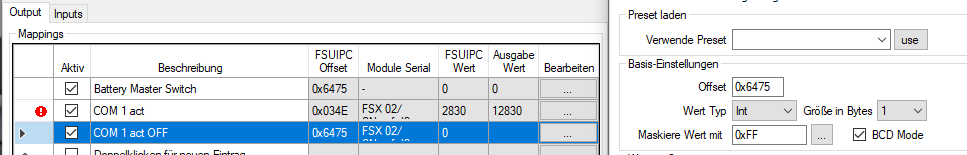Ich erstelle eine neue Config, die ich COM1 act OFF nenne. Im Tab FSUIPC wähle ich den Battery Master Switch aus, da ich diesen ja überwachen möchte.

Nun wechsele ich in den Tab „Vergleich“ und aktiviere ihn mit dem Haken.

Beim aktuellen Wert möchte ich nun, daß der Vergleich aktiv wird, wenn der Wert des Battery Master Switches 0 ist. Das trage ich in die 1. Zeile ein.

In die Zeile „dann setze“ trage ich 5 Leerzeichen ein. Daher sind diese auf dem Screenshot nicht sichtbar!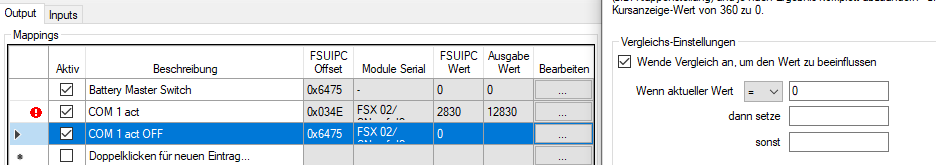Jetzt in den Tab Display wechseln und den Haken in der Zeile „set decimal point“ entfernen, sonst würde dieser bei einem leeren Display trotzdem angezeigt werden.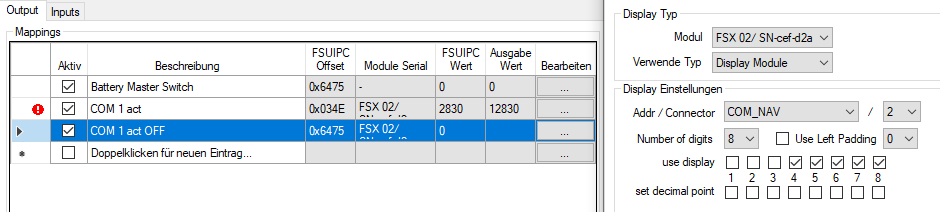Als Letztes in den Tab Vorbedingung wechseln und hier wieder den Haken vor die Vorbedingung setzen. Wieder den Config Eintrag auswählen und darunter die Config Battery Master Switch. Diesmal bei „wenn aktueller Wert“ „=“ auswählen und daneben eine 0 eintragen und auf „Anwenden“ klicken. Alles mit OK bestätigen und fertig.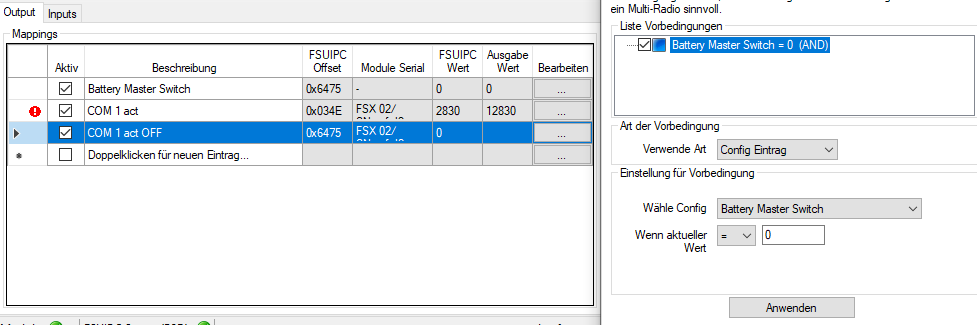Wie im Screenshot erkennbar, ist der Battery Master Switch ausgeschaltet, somit ist sein Offset 0, das Com1 Display ist inaktiv, dessen Ausgabe wird geblocked (rotes Ausrufezeichen) und die COM1 act OFF Konfiguration ist aktiv und gibt keine Ausgabe aus. Tatsächlich werden 5 Blanks ausgegeben, aber das ist so nicht sichtbar. Wer es nicht glaubt, setzt in den Vergleich eine beliebige Zahl ein.

Schalte ich den Battery Master Switch nun ein, so passiert folgendes:

Der Wert des Offsets wechselt von 0 auf 1, die COM1 act Config wird aktiv und die COM1 act OFF Config wird durch das rote Ausrufezeichen deaktiviert und die Frequenz wird auf dem Display angezeigt: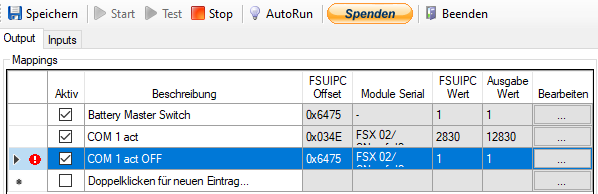Der erfahrene User kann die Config „COM 1 act OFF“ auch ohne Offset 0x6475 schreiben, weil durch die Vorbedingung (BATTERY MASTER SWITCH=0) dieser Offset bereits überwacht wird.

Dies war Teil 3 des Guides über Formeln, der sich mit Vorbedingungen beschäftigt hat.  Teil 4 beinhaltet den Umgang mit der Interpolation.

Pizman82 und StephanHo für MobiFlight im Dezember 2020